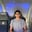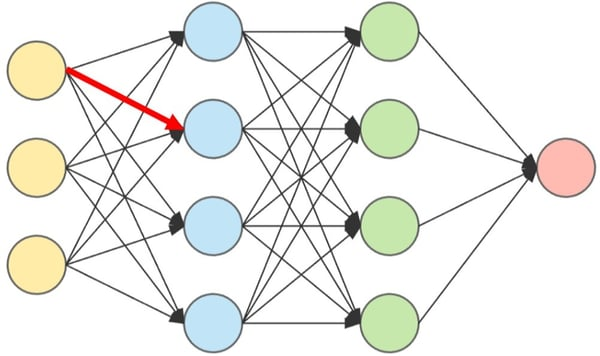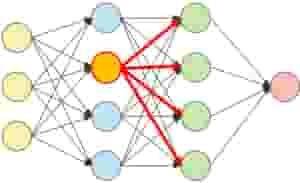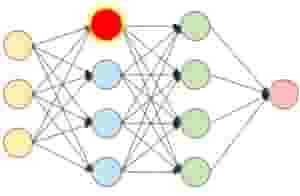Related Tags

batch
normalization

# What is batch normalization?Taimur Hassan Sarmad

### Overview

To understand what batch normalization is, we first need to discover why the use of simple normalization alone doesn't suffice.

### Why isn’t simple normalization enough?

Even if we employ normalization to our data, another problem arises when one of the weights becomes drastically larger than the other weights in the neural network during the training phase.One weight (in red) becomes much larger than all the other weights

When this weight cascades down through the other layers in the network, the gradients become unbalanced. This leads to the exploding gradientWhen the backpropagation algorithm is unable to make any meaningful update to the weights because of the gradient (which is used to update the weights) growing exponentially problem.The neuron receiving the significantly larger input (colored yellow with a red border) produces a comparatively much larger output which goes into all the neurons in the next layer

This makes the neural network highly unstable, effectively rendering it incapable of learning anything from the training data.

The main reason behind the problem described above is the activation function. Whenever a linear (or rectified linear) activation function is employed, the output can take any possible value. Since linear activation functions don’t bound the output, during the training process, a point may arise where the output becomes drastically large.

Since activation functions are used by each neuron in every layer, at least one of them may be a linear/rectified linear function. Hence, simple normalization techniques can’t solve this issue (since these only deal with the inputs provided to the input layer, whereas this issue arises in the hidden layers), and this is where batch normalization comes into play.

### Batch normalization

Batch normalization can be applied to all the layers in the neural network. Alternatively, we can choose the specific layers to apply it to.

During the process of batch normalization, the weights are updated after each batchThe input data is divided into smaller groups called ‘batches’, and each epoch involves passing all these ‘batches’ through the network once. We do this division because feeding all the data at once can really slow the network down. of m samples is seen by a layer (and hence, the network is trained by mini-batch gradient descent).

To illustrate the math behind batch normalization, let’s consider an example with a batch size of three (m=3) and focus on the following neuron:We'll focus on the neuron in red for now. The weights of this neuron will be updated after three training samples have been seen by it

Next, let’s suppose that we observe the following:

• After the first sample is passed, our neuron has an activation $a_1$ of $4$.
• After the second sample is passed, our neuron has an activation $a_2$ of $7$.
• After the third sample is passed, our neuron has an activation $a_3$ of $5$.

Since three samples have now been observed, the entire batch has been seen and now our neuron can pass its outputs ahead to the next layer to complete the forward pass step and eventually begin the backward propagation. However, instead of just passing the activations as they are, we will now be applying batch normalization and modifying the activations.

First, we standardize (using the method described above) all the activations for this particular batch by the batch mean and standard deviation.

The mean of the activations for this batch is $5.33$, and the standard deviation of this batch’s activations is $1.247$. Thus, the standardized activations are the following:

$a_1'=-1.068$

$a_2'=1.341$

$a_3'=-0.265$

where $a_i'$ is the standardized activation for the $i$th sample.

These activations for the batch now lie within a much smaller range. They also have a zero mean and a unit standard deviation.

This modification of activations is done for each neuron in the layer.

The process is still not complete, as there is still a glaring loophole—the mean and standard deviation depend heavily on the samples in each batch, and so the range of the activations/outputs at one layer might still vary from the activations/outputs at another layer to a great extent. To deal with this, we introduce two arbitrary parameters—$\gamma$ and $\beta$—such that $y_i=\gamma a_i+\beta$ , where $y_i$ is the activation for the $i$th sample in the batch.

These newly introduced parameters are learnableLearnable parameters are adjusted during the training phase by the neural network itself - like the weights and biases (these are examples of learnable parameters).. When trained on multiple batches, $\gamma$ approaches the true mean of all the activations of our neuron (and not just the mean of the activations caused by a particular batch), and $\beta$ approaches the true standard deviation of all the activations of our neuron. This way, each neuron in the neural network will have its own $\gamma$ and $\beta$ and all these neurons will produce activations $y$ which lie in a similar range. Once these activations are produced, they are passed onto the next layer to continue the forward pass step of the neural network.

Thus, batch normalization helps overcome the problem of exploding gradients by keeping all the activations within a uniform scale, and this scaling also speeds up the training process!

The executable code snippet below demonstrates batch normalization at work. It employs just the input layer and the first hidden layer from the above example.

from numpy.random.mtrand import normal
import numpy as np
import math
import matplotlib.pyplot as plt
import scipy.stats as stats

# example neural network:

# number of input features = 3
# number of input samples = 12
# number of hidden layers = 1
# number of neurons in hidden layer = 4

batch_size = 3
number_of_input_samples = 12
number_of_features = 3
input_samples = []
num_of_hidden_neurons = 4

for i in range(0,number_of_input_samples): # Making 12 input samples, with each input having 5 features.
inputs = np.random.randint(1 , 11 , size = number_of_features) # providing random input values between 1 and 10
input_samples.append(inputs)

xavier_weights = np.random.standard_normal(size=(5,10))*(1/(number_of_features**0.5)) # xavier initialization of weights
xavier_weights = 15 # instead of implementing an entire neural
# network with back-propagation and gradient descent, we will simulate
# one by hard-coding the weight from the first input feature to the first
# neuron in the hidden-layer to be a large value (and assume that this value
# became large during some stage in the training process).

def activation(z):
return max(0 , z)

# finding the z at each neuron in the hidden layer (with Xavier Initialization):
normalized_batch_activations = []
neuron_activations = []

# WITHOUT BATCH NORMALIZATION:
og_activations = []
current_batch_activations = []
for sample in range(0,number_of_input_samples):
neuron_activations = []
for i in range(0,num_of_hidden_neurons):
z = 0
for j in range(0,number_of_features):
z = z + xavier_weights[j][i]*input_samples[sample][j]

neuron_activations.append(activation(z))

current_batch_activations.append(neuron_activations)

if len(current_batch_activations) == batch_size:
og_activations.append(current_batch_activations)
current_batch_activations = []

og_activations = np.array(og_activations)
print("Activiation of first neuron in hidden layer: " , og_activations)

# WITH BATCH NORMALIZATION:
current_batch_activations = []
for sample in range(0,number_of_input_samples):
neuron_activations = []
for i in range(0,num_of_hidden_neurons):
z = 0
for j in range(0,number_of_features):
z = z + xavier_weights[j][i]*input_samples[sample][j]

neuron_activations.append(activation(z))

current_batch_activations.append(neuron_activations)

if len(current_batch_activations) == batch_size:
current_batch_activations = np.array(current_batch_activations)
batch_mean = np.mean(current_batch_activations , axis=0)
batch_dev = np.std(current_batch_activations, axis=0)
for i in range(0,batch_size):
for j in range(0, num_of_hidden_neurons):
current_batch_activations[i][j] = (current_batch_activations[i][j] - batch_mean[j])/(batch_dev[j]+1)

normalized_batch_activations.append(current_batch_activations)
current_batch_activations = []

normalized_batch_activations = np.array(normalized_batch_activations)
print("\nActiviation of first neuron in hidden layer (with Batch Normalization): " , normalized_batch_activations)
It can be seen that without batch normalization, the neuron's activation is much larger.

RELATED TAGS

batch
normalization

CONTRIBUTORTaimur Hassan Sarmad
RELATED COURSES

View all Courses

Keep Exploring

Learn in-demand tech skills in half the time